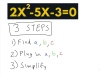#### What do you want to calculate?

Example: 2x^2-5x-3=0

### Step-By-Step Example

Learn step-by-step how to use the quadratic formula!

### Example (Click to try)

Solve an equation of the form ax2+bx+c=0 by using the quadratic formula:
x=
 −b±√b2−4ac 2a

### Step-By-Step Video Lesson